# 35 Results

View
Selected filters:
• Desmos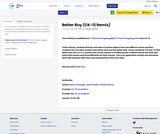Unrestricted Use
CC BY
Rating

In this activity, students find the unit rates of various objects from two different stores and then compare the unit rates to determine which store has the better deal.

Subject:
Patterns, Functions, and Algebra
Material Type:
Activity/Lab
Author:
Sarah Leahey
12/18/2019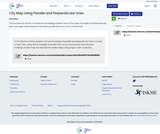Unrestricted Use
CC BY
Rating

This is a Desmos activity to assess the knowledge students have of the slope of parallel and perpendicular lines and angle relationships formed when parallel lines are cut by a transversal.

Subject:
Measurement and Geometry
Material Type:
Interactive
Author:
Kaitlin Bookbinder
12/11/2020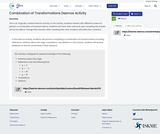Unrestricted Use
CC BY
Rating

This is an originally created Desmos activity. In this activity, students interact with different screens to perform a combination of transformations. Students will check their work and, upon revealing the answer, will not be able to change their answers. After checking their work, students will justify their creations.

Subject:
Measurement and Geometry
Material Type:
Interactive
Author:
Kaitlin Bookbinder
11/16/2020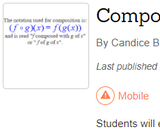Unrestricted Use
CC BY
Rating

Students evaluate functions to make a connection to compositions of functions.  Students then practice finding compositions of functions.  Students end the activity evaluating compositions of functions both algebraically and graphically with a challenge that includes multiple compositions.

Subject:
Algebra I & II
Material Type:
Activity/Lab
Author:
Candice Barkley
12/11/2020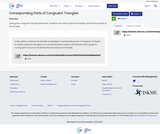Unrestricted Use
CC BY
Rating

Using given congruent triangle statements, students will create congruent triangles and find the lengths of all the sides.

Subject:
Measurement and Geometry
Material Type:
Interactive
Author:
Kaitlin Bookbinder
02/08/2021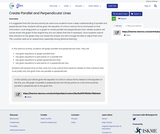Unrestricted Use
CC BY
Rating

It is suggested that this activity be used once students have a deep understanding of parallel and perpendicular lines. Students will be given the equation of a line in various forms and based on that information, must drag points on a graph to create parallel and perpendicular lines. Initially students will not be shown the graph of the original line, but can obtain that hint if necessary. Once students submit their solutions on the graph, they can reveal the answer, but will no longer be able to adjust their work. This could be used as an assessment, especially during distance learning.

Subject:
Measurement and Geometry
Material Type:
Activity/Lab
Homework/Assignment
Interactive
Author:
Kaitlin Bookbinder
11/16/2020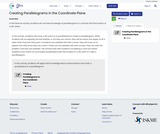Unrestricted Use
CC BY
Rating

In this Desmos activity, students will use their knowledge of parallelograms to correctly find the location of a 4th vertex.

Subject:
Measurement and Geometry
Material Type:
Interactive
Author:
Kaitlin Bookbinder
03/11/2021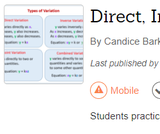Unrestricted Use
CC BY
Rating

Students practice identifying the types of variation from equations and solving problems of different types of variation.

Subject:
Algebra I & II
Material Type:
Activity/Lab
Author:
Candice Barkley
02/18/2021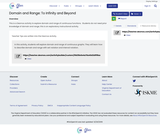Unrestricted Use
CC BY
Rating

This is a Desmos activity to explore domain and range of continuous functions.  Students do not need prior knowledge of domain and range; this is an exploratory instructional activity.

Subject:
Algebra I & II
Material Type:
Interactive
Author:
Javier Cabezas
12/11/2020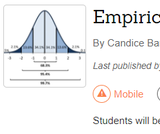Unrestricted Use
CC BY
Rating

Students will be introduced to the Empirical Rule for normal distributions and practice using it to answer questions.

Subject:
Algebra I & II
Material Type:
Activity/Lab
Author:
Candice Barkley
02/18/2021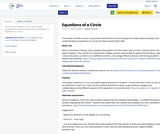Unrestricted Use
CC BY
Rating

This resource provides a warm-up, concept development, practice and alternate assessment for Equations of a Circle.

Subject:
Measurement and Geometry
Material Type:
Activity/Lab
Assessment
Homework/Assignment
Author:
Rebecca Hall
01/04/2020Unrestricted Use
CC BY
Rating

Students evaluate functions with function notation both algebraically and graphically.

Subject:
Algebra I & II
Material Type:
Activity/Lab
Author:
Candice Barkley
02/18/2021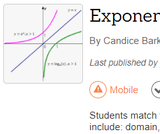Unrestricted Use
CC BY
Rating

Students match features of exponential and logarithmic functions and then consider which features could change with a transformation. Features include: domain, range, intervals of increasing/decreasing, x- and y- intercepts, end behavior, and asymptotes.

Subject:
Algebra I & II
Material Type:
Activity/Lab
Author:
Candice Barkley
02/18/2021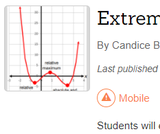Unrestricted Use
CC BY
Rating

Students review domain, range, and intervals of increasing and decreasing using a draggable point to help them find extrema.  Students find both absolute and relative extrema.

Subject:
Algebra I & II
Material Type:
Activity/Lab
Author:
Candice Barkley
11/25/2020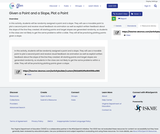Unrestricted Use
CC BY
Rating

In this activity, students will be randomly assigned a point and a slope. They will use a movable point to plot a second point and receive visual feedback via animation as well as explicit written feedback about the slope of the line they created. All starting points and target slopes are generated randomly, so students in the class are not likely to get the same problems within a slide. They will all be practicing plotting points given a slope.

Subject:
Mathematics
Algebra I & II
Material Type:
Activity/Lab
Homework/Assignment
Author:
Javier Cabezas
02/09/2021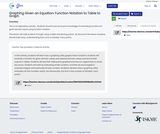Unrestricted Use
CC BY
Rating

This is an exploratory activity.  Students should have some prior knowledge of evaluating functions for given domain inputs using function notation.This lesson will walk students through using a table and plotting points.  By the end of the lesson students should walk away understanding that a line is infinitely many points.

Subject:
Mathematics
Material Type:
Interactive
Author:
Javier Cabezas
12/11/2020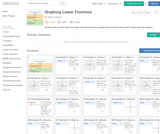Conditional Remix & Share Permitted
CC BY-NC
Rating

This is a guided practice, instructional activity. First students create input-output tables to graph. Then students apply slope-intercept reasoning to graph linear equations. Equations are given in slope-intercept form.

Subject:
Algebra I & II
Material Type:
Activity/Lab
Author:
Kelly Johnson
02/01/2021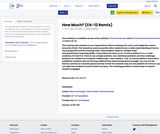Unrestricted Use
CC BY
Rating

This activity asks students to use proportions to find a missing unit cost or unit weight for various amounts of fruit. This should be used as practice after students have a solid understanding of how to use a proportion to find a missing value.

Subject:
Computation and Estimation
Material Type:
Activity/Lab
Author:
Sarah Leahey
12/18/2019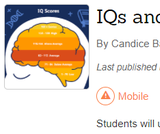Unrestricted Use
CC BY
Rating

Students will use IQ data to practice finding and interpreting z-scores.

Subject:
Algebra I & II
Material Type:
Activity/Lab
Author:
Candice Barkley
01/18/2021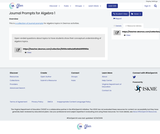Unrestricted Use
CC BY
Rating

This is a collection of journal prompts for algebra topics in Desmos activities.

Subject:
Algebra I & II
Material Type:
Assessment
Author:
Javier Cabezas